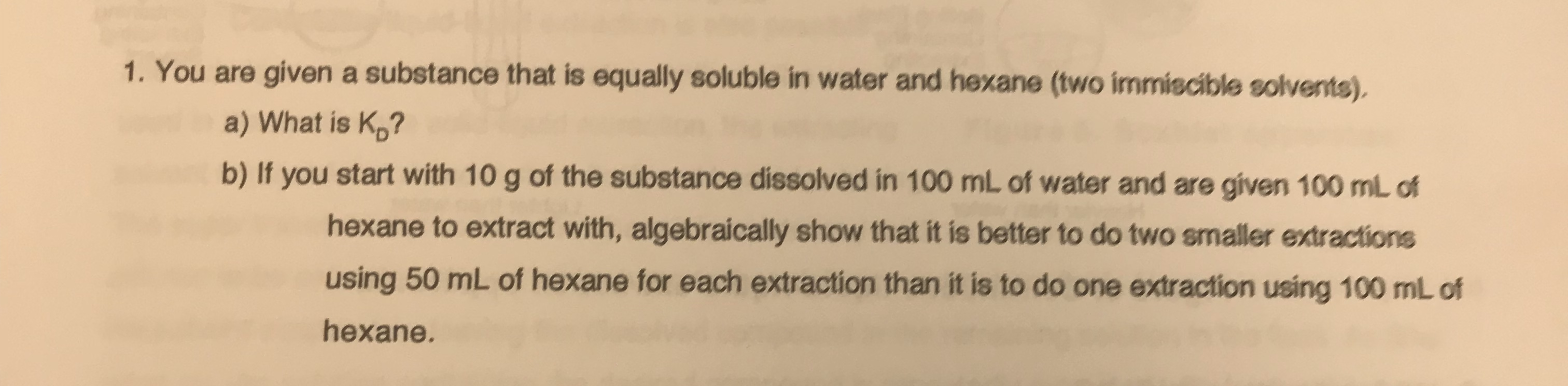# 1. You are given a substance that is equally soluble in water and hexane (two immiscible solvents).a) What is Kp?b) If you start with 10 g of the substance dissolved in 100 mL of water and are given 100 mL ofhexane to extract with, algebraically show that it is better to do two smaller extractionsusing 50 mL of hexane for each extraction than it is to do one extraction using 100 mL ofhexane.

Question
82 viewshelp_outlineImage Transcriptionclose1. You are given a substance that is equally soluble in water and hexane (two immiscible solvents). a) What is Kp? b) If you start with 10 g of the substance dissolved in 100 mL of water and are given 100 mL of hexane to extract with, algebraically show that it is better to do two smaller extractions using 50 mL of hexane for each extraction than it is to do one extraction using 100 mL of hexane. fullscreen
check_circle

Step 1

Water is a polar solvent while hexane is non-polar in nature. Due to this, the two solvents are immiscible and form two layers when mixed together.

Hexane, being lighter forms the top layer while water forms the bottom one.

If a substance to be extracted is equally soluble in both these solvents, then it will distribute itself between the two layers in such a way that its concentration is equal in both the solvents.

Step 2

a) The ratio of concentration of substance in the system of two immiscible solvents is called partition coefficient or distribution coefficient. It is denoted by Kd

For present case, the substance is equally soluble. So, its concentration in hexane will be equal to its concentration in the water layer.

Hence, Kd can be calculated by taking ratio of Concentration in hexane : Concentration in water.

As concentrations are equal, Kd = 1

Step 3

b) As Kd = 1, when 10 g of substance would be added to the system  containing  100 mL each of hexane and water.

To, equalize its concentra...

### Want to see the full answer?

See Solution

#### Want to see this answer and more?

Solutions are written by subject experts who are available 24/7. Questions are typically answered within 1 hour.*

See Solution
*Response times may vary by subject and question.
Tagged in

### Chemistry